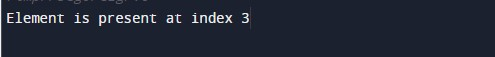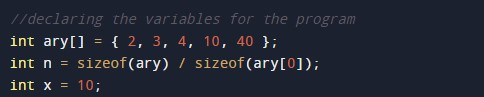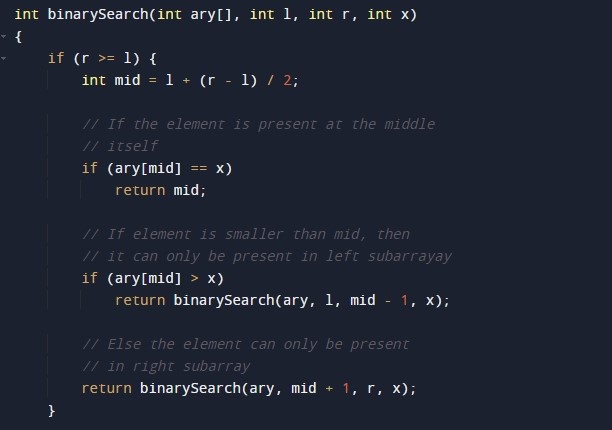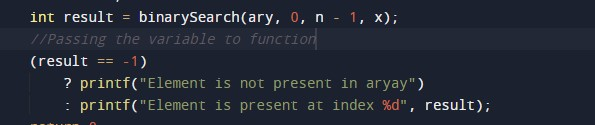C Program to Implement Binary Search

In this tutorial you will learn about the C Program to Implement Binary Search and its application with practical example.

C Program to Implement Binary Search

In this tutorial, we will learn to create a C program that will do Implement Binary Search using C programming.

Prerequisites

Before proceeding with this tutorial we assume that you are best aware of the following C programming topics:

• Operators in C Programming.
• Basic Input and Output function in C Programming.
• Basic C programming.
• Conditional statement in C Programming.
• Creating and Using the user-defined function in C programming.

Implement Binary Search

In every programming language, the sorting of data is a very important factor. Sorting works are done mainly with the techniques available in C Language. Many different techniques for sorting are available to work.

But the data structure work is incomplete without the searching of the data. In today’s tutorial, we will implement the binary search in the array to find the element in the array.

Output:-In the above program, we have first declared and initialized a set of variables required in the program.• n = it will hold the size of an array.
• ary[]= it will hold the elements in an array.

After this, we make the user-defined function for searching.Printing the output.In this tutorial we have learn about the C Program to Implement Binary Search and its application with practical example. I hope you will like this tutorial.